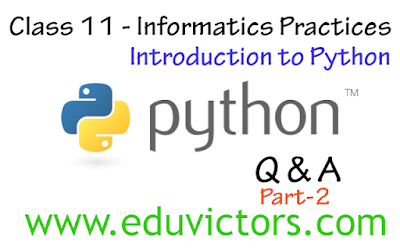## Pages

Showing posts with label class11-Informatics Practices. Show all posts
Showing posts with label class11-Informatics Practices. Show all posts

# Class 11 Informatics PracticesIntroduction to Databases Questions and Answers on MySQL (Part 1)

Q1. What is database?

Answer: A database is an organized collection of data. The data are typically organized to model aspects of reality in a way that supports processes requiring this information. The term "database" can both refer to the data themselves or to the database management system.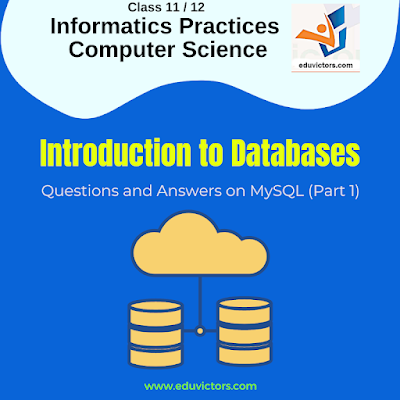# Class 11 - Informatics Practices - Python Snippets (Set-2)

Write Python Programs for the following problem statements:

1. Write a program in python to input marks in 5 subjects from the user and display the average marks.

2. Write a program in python to read details like name, class, age of a student and then print the details firstly in same line and then in separate lines.

3. Write a program in python to input a number and print its first five multiples.

4. Write a program to read a number n and print n, n² , n³ and n⁴

5. Write a program that generates the following output: 5 @ 10 @ 9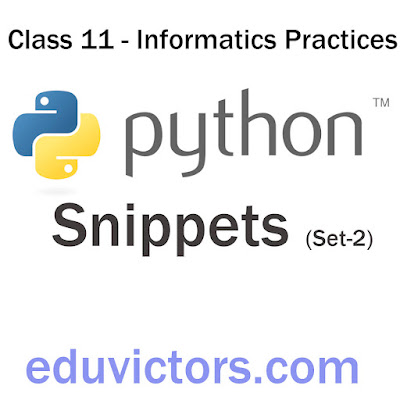# Class 11 - Informatics Practices - Emerging Trends (Worksheet)

Fill in the blanks with a suitable word from the list given below.

AI, Augmented reality, Big Data, Blockchain, Cloud computing, Data Analytics, IoT, Machine Learning(ML), Natural Language Processing (NLP), Robotics, Sensors, Virtual Reality (VR)

1. ________ endeavours to simulate the natural intelligence of human beings into machines thus making them intelligent.

2. This field of AI comprises algorithms that use data to learn on their own and make predictions. We are talking about ______________.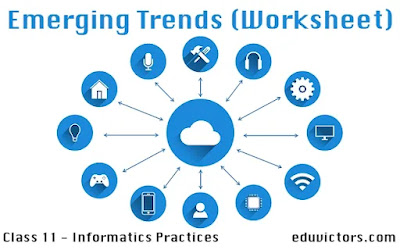# CBSE Class 11 - Python Conditional Statements - Computer Science/ Informatics Practices

Q1: What do conditional statements do?

Answer: Conditional statements let us write program to do different tasks or take different paths based on the outcome of the conditions.

Q2: What is meant by a control structure?

Answer: A control structure is a logical design that controls the order in which a set of statements execute.

Thre are three types of control structures:# CBSE Class 11 - Informatics Practices - Brief Overview Of Python (MCQs)

Q1: Who developed Python language?

(a) Bill Gates

(b) Guido Van Rossum

(c) Jeff Bezos

(d) Steve Jobs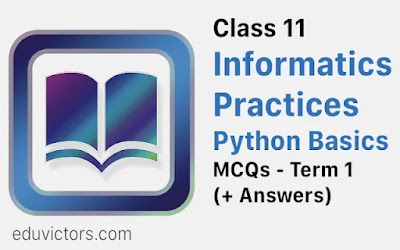# CBSE Class 11 - Informatics Practices - Computer System (MCQs)

Q1: Which of the following is called brain of the computer?

(a) Keyboard

(b) Computer screen

(c) Processor

(d) Printer

Q2: Which of the following is not the physical component of a computer system?

(a) Primary Memory

(b) Computer Software

(c) CPU

(d) Output Device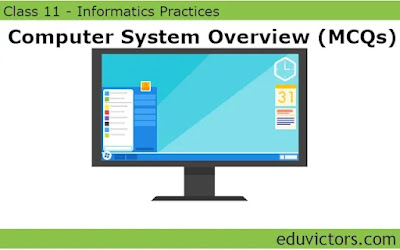# Worksheet on Database Management Systems

Class 10 - Information Technology

Class 11/Class 12 - Computer Science / Informatics Practices

Directions: Fill in the blanks with suitable words.

1 _______ is a collection of related information.

2 _______ are the basic building blocks of a database.

3 Table is also known as ________

4 In a table, columns are also called ________ and rows are called _________.## Computer System Overview

(Part-2)
Class 11 - Informatics Practices / Computer Science
Q1: What is an input device?

Answer: A device through which data and programs from the outside world enter the computer system is called an input device. It  converts the input data into a digital form that is acceptable by the computer system.

Q2: Identify the input device from the picture given below. Write one line description about each item .## Computer System Overview

(Part-1)
Class 11 - Informatics Practices / Computer Science

Q1: What is a computer?

Answer: A computer is an electronic device, under the control of instructions stored in its memory that can accept data (input), process the data according to specified rules(Program), produce information (output), and store the information for future use.

The word 'computer' comes from the word 'compute' which means to calculate.  Therefore, a computer is an electronic device that can perform arithmetic operations at high speed.

Q2: Why a computer is also called a data processor?

Answer: A computer is also called a data processor because it can store, process and retrieve data whenever desired.## Numpy Arrays (Part-1) - Question and Answers

CBSE Class 11 - Informatics Practices - Python Basics

Q1: The following statement has an error. Write the correct statement.
>>> import numpy as np
>>> a = np.array(1,2,3,4)

Answer: The second statement should be a = np.array([1,2,3,4]) to create an ndarray.

Q2: What is the output of the following program?

import numpy as np
list1 = [1,2,3,4,5]
np1 = np.array(list1)
print(type(np))
print(type(np1))
print(np1)
print(np1.shape)

<class 'module'>
<class 'numpy.ndarray'>
3
(5,)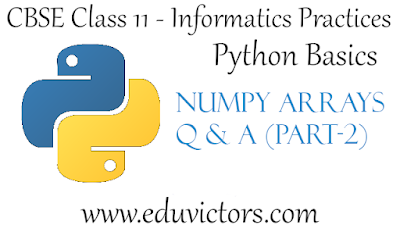## Python Basics - Numpy Arrays

CBSE Class 11 - Informatics Practices

Q1: What is NumPy?

1. NumPy stands for Numerical Python.

2. It is the core library for scientific computing in Python.

3. A NumPy array (also called ndarray) is a homogeneous multidimensional array of data objects.

4. A NumPy array is a table of elements (usually numbers), all of the same type, indexed by a tuple of positive integers.

5. Numpy module provides a set of methods and tools for working with these arrays.

6. In Numpy dimensions are called axes. The number of axes is rank.

Q2: What is the command line option to install NumPy module?

Answer: Run the following command at the command prompt.
pip install numpy

Q3: What is one dimensional (1D) array?

Answer: A one-dimensional array or 1D array is a named group of a contiguous set of elements having the same data type. 1D arrays are also called vectors.

Q4: What is a multi-dimensional array?

Answer: A multidimensional array is an array of arrays. Multi-dimensional arrays are also known as matrices. For example, a two-dimensional array (2D array) has two axes i.e. rows and columns.## Python Basics - List Manipulation (Part-2) - Question and Answers

CBSE Class 11 - Informatics Practices

Based on syllabus of Class 11 Informatics Practices, here are important Questions and Answers covered for the chapter List Manipulation.

The questions and answers will familiarise you with basics concepts of Python Lists and provide a framework for understanding the programming concepts.Software development isn’t any one thing, but a myriad of practices that coalesce into software in the end. Python has been a versatile language and is used by companies to write all sorts of applications.

List is an important data structure, part of a core Python. Lists have a variety of uses including data science, statistics and scientific computing. List is considered as a collection of objects and is ordered and changeable(mutable). It allows duplicate members.

Q1: What is the output of the following code?

list =['I','N','D','I','A']
print(list[0:3])
print(list[3:])
print(list[:])

['I', 'N', 'D']
['I', 'A']
['I','N','D','I','A']

Q2: What is the output of the following code? What does the '+' operator' do in the code snippet?## Python Basics - List Manipulation (Part-1)

CBSE Class 11 - Informatics Practices & Computer Science

Q1: What is a list in Python?

Answer: List is a collections of items and each item has its own index value. It is the most versatile datatype available in core Python which can be written as a list of comma-separated values (items) between square brackets.

e.g.
[] # empty list
[1,2,3,4] #list of integers
[2.0, 4.56, 8.91, ] #list of floats
['Sunday', 'Monday', 'Tuesday'] #list of floats
['Nikita', 16, 'Class 11', True, 86.7] # list of mixed values## Python - String Manipulation (Part-2)

CBSE Class 11 - Informatics Practices

Q1: What are membership operators for strings?

in Returns True if a substring exists in the given string otherwise returns false.
e.g. "xy" in "xyz"  #returns True
"ab" in "xyz"  #returns False

not in Returns True is a substring does not exist in the given string;
e.g. "ab" not in "xyz"   #returns True
"xy"  not in "xyz"   #returns False

Q2: What is the output of the following?
str1 = "Hello World!"
str2 = "he"
str3 = "He"
str2 in str1
str3 in str1## Python Basics - Type Conversion and Type Casting

CBSE Class 11 - Informatics Practices

Question 1: What is Type Conversion?

Answer: Type conversion means converting one data type to another data type. Python supports two types of type conversion:

1. Implicity Type Conversion
2. Explicit Type Conversion (also called Type Casting)

Question 2: What is implicit type conversion? Give an example.## Python - String Manipulation (Part-1)

CBSE Class 11 - Informatics Practices

Q1: What is a string in Python?

Answer: String is a sequence of characters, which is enclosed between either single(' ') quotes or double quotes(" "), python treats both single and double quotes same.
e.g.
str1 = "Welcome to the Python World."

Q2: Can a double-quoted string contain single quotes? If yes, give an example.

Example:
str1 = "Nitika's Fashions"
print(str1)

Similarly, A single-quoted string can also contain double quotes:
Example:
str2 = '"Help!", he exclaimed.'
print(str2)## Python - Augmented Assignment Operators

CBSE Class 11 - Informatics Practices

Python provides augmented assignment operators, which combine the impact of an arithmetic operator with an assignment operator.

e.g. If you want to add value of b to a and then assign the result to a i.e.  a = a + b can be written as
a += b

Various augmented operators are:

OperationDescription
x += yx = x + y
x -= yx = x - y
x *= yx = x * y
x /= yx = x / y
x //= yx = x // y
x **= yx = x ** y
x %= yx = x % y
x &= yx = x & y
x |= yx = x | y
x ^= yx = x ^ y
x >>= yx = x >> y
x <<= yx = x << y## CBSE Class 11 - Informatics Practices

Question: What is a character set? What is Python Character Set?

Answer: A set of valid characters recognized by python constitutes character set.

Python (from version 3.x onwards) supports the Unicode text. It has the following character set:
Letters: A-Z,a-z
Digits:  0-9
Special symbols: Special symbol available over a keyboard
White spaces: blank space,tab, carriage return, newline, form feed
Other characters: Unicode

Question: Can I type in Hindi in Python 3?

Answer: Yes, Python supports Unicode text, hence one can use multiple languages including Hindi.

Question: What is a token?

Answer: Smallest individual unit in a program is known as a token. It can be:
1. Keywords
2. Identifiers
3. Literals
4. Operators
5. Punctuators## Class 11 - Informatics Practices - Python - Variables

Q1: What are variables?

Answer: A variable is a name that is used to store data. It can be used to store different kinds of data.
e.g. age = 16

It defines a variable named 'age' and stores integer value as '16'. Thus 'age' is an integer data type variable.

Every variable in Python is 'dynamic type' i.e. you need not declare data type of a variable or declare a variable before using it. Every variable in Python is an object.

Q2: What are the rules to define a valid variable?## Python Basics  Q & A (Part-2)

CBSE Class 11 - Informatics Practices

Q1: What are the different modes that can be used to test Python Program?

Answer: In Python, programs can be written in two ways namely Interactive mode and Script mode.
Interactive mode or Interpreter mode allows us to write codes in Python command prompt  (  >>> ).
Script mode is used to create and edit python source file with the extension .py

Q2: Write the command to leave python shell or interpreter.

Answer: ^D (Ctrl+D) or quit () is used to leave the interpreter.

Q3: What is the advantage of interpreter mode?

Answer:  Working in interactive mode is convenient for beginners and for testing small pieces of code, as we can test them immediately.